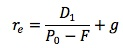Subject 7. Flotation Costs
#cfa #cfa-level-1 #corporate-finance #cost-of-capital #has-images
Flotation costs are the costs of issuing a new security, including the money investment bankers earn from the spread between their cost and the price offered to the public, and the accounting, legal, printing and other costs associated with the issue.

The amount of flotation costs is generally quite low for debt and preferred stock (often 1% or less of the face value), so we ignore them here. However, the flotation costs of issuing common stocks may be substantial, so they must be accounted for in the WACC. Generally, we calculate this by reducing the proceeds from the issue by the amount of the flotation costs and recalculating the cost of equity.Example 1

XYZ is contemplating issuing new equity. The current price of their stock is $30 and the company expects to raise its current dividend of$1.25 by 7% indefinitely. If the flotation cost is expected to be 9%, what would be the cost of this new source of capital?

Cost of external equity = (1.25 x 1.07) / (30 x (1 - 0.09)) + 0.07 = 11.9%

Without the flotation cost, the cost of new equity would be (1.25 x 1.07) / 30 + 0.07 = 11.46%.

Note that flotation costs will always be given, but they may be given as a dollar amount or as a percentage of the selling price.

This is a typical example found in most textbooks. One problem with this approach is that the flotation costs are a cash flow at the initiation of the project and affect the value of any project by reducing the initial cash flow. It is not appropriate to adjust the present value of the future cash flows by a fixed percentage. An alternative approach is to make the adjustment to the cash flows in the valuation computation.

Example 2

Continue with the above example. Assume that XYZ is going to raise $10 million in new equity for a project. The initial investment is$10 million and the project is expected to produce cash flows of $4.5 million each year for 3 years. Ignoring the flotation cost of issuing new equity, the NPV of the project will be -10 + 4.5/1.11461 + 4.5/1.11462 + 4.5/1.11463 =$0.9093 million.

Now consider the flotation cost of 9%. The NPV, considering the flotation costs, is 0.9093 - 0.9 = $0.0093 million. However, if we use the "typical" approach, the NPV. considering the flotation costs, will be -10 + 4.5/1.1191 + 4.5/1.1192 + 4.5/1.1193 =$0.8268 million.
If you want to change selection, open original toplevel document below and click on "Move attachment"